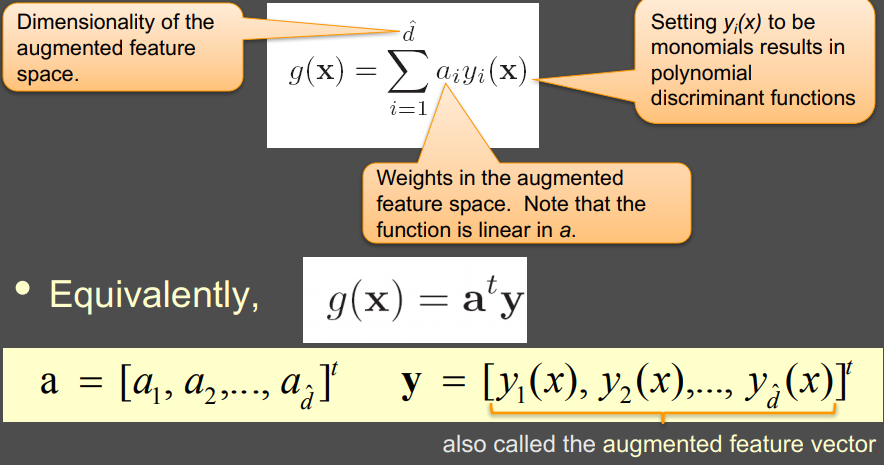5 views

With a single-layer perceptron, it's easy to find the equation of the "separating line" (I don't know the professional term), the line that separates between the 2 types of points, based on the perceptron's weights, after it was trained. How can I find similarly the equation of the curve (not a straight line) that separates between the 2 types of points, in a multi-layer perceptron?

thanks.

by (108k points)

Generalized Discriminant Function:Let y1,y2,…,yn be n training samples in augmented feature space, which is linearly separable

• We need to find a weight vector such that:

1.  a^t y > 0 for examples of the positive class
2.  a^t y < 0 for examples of the negative class

• “Normalizing” the input examples by multiplying them with their class label (replace all samples from class 2 by their negatives) find a weight vector such that a^t y > 0 for all the examples (here y is multiplied with class label)

• The resulting weight vector is termed as a separating vector or a solution vector